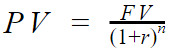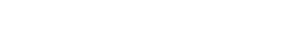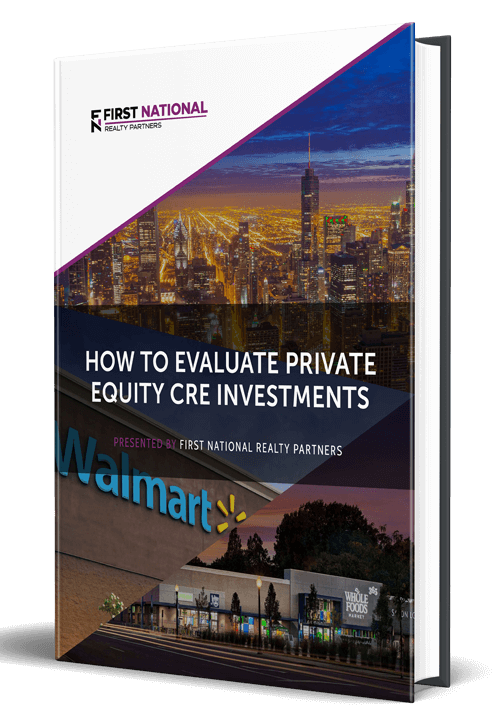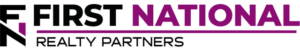# Understanding Present Value and Net Present Value

### Key Takeaways

• The basic premise of a commercial real estate investment is that a sum of money is paid upfront to purchase a property with the expectation that it will be returned, with a profit, in a series of future annual cash flows.
• It can be difficult to value a series of cash flows because they occur over a multi-year time period and the “time value of money” concept dictates that money received today is worth more than money received in the future due to its ability to be invested and earn interest.
• To account for this concept, Present Value and Net Present Value are two metrics that take anticipated cash flows and “discount” them back to the present time using a chosen return requirement.
• The result of these calculations can provide valuable insight into the best purchase price for a property and the ultimate profitability of an investment.

The basic premise of a commercial real estate investment is relatively simple.  An investor purchases a property and leases the space in it to other individuals or businesses.  Lease income is used to pay the property’s operating expenses and any remaining funds are distributed back to the property owners.  Over a multi-year investment period, the return can be significant, but it becomes a challenge to model because a dollar earned 5 or 8 years in the future does not necessarily have the same value as a dollar earned today.  This is a concept known as the time value of money and it is a critical component behind the Present Value and Net Present Value calculations.

## What is the Time Value of Money?

The concept of the time value of money is the idea that a dollar earned today is worth more than a dollar earned in the future due to its ability to be invested and earn interest.  For example, if an investor has a choice between receiving \$5,000 today or \$5,000 in five years, the rational choice would be to take the money today because it could be invested and the resulting return could make the sum worth more than the \$5,000 that would be received in five years.

This concept is relevant for a commercial real estate investment because property cash flows are earned over a multi-year time period.  As such, it is necessary to “discount” future cash flows back to the present time when attempting to calculate their “present value.”

## Present Value – Defined

Remember the basic premise behind a commercial real estate investment is that an initial investment is made today to purchase a property, which is expected to deliver some series of future cash inflows.  Using the time value of money concept as a guide, it reasons that an investor would expect to earn some return on their investment as compensation for waiting to receive the future cash flows.  This required return is a measure of their personal assessment of the risk that they will actually receive it.  So, for example, if one investor determines the risk to be high, they may demand a return of 15% or if another determines the risk to be low, they may be fine with a return of 5%.  When calculating the present value of a series of cash flows, this rate is often referred to as the “discount rate.”

So, the Present Value (PV) is the current value of a series of future cash flows given a defined rate of return, i.e. the discount rate.  The formula for calculating it is relatively simple, it is:In the formula, FV is the anticipated future cash flow, r is the required rate of return, and n is the number of periods (years) for which the cash flow must be discounted.  In other words, a property’s present value is simply the sum of expected future cash flows discounted back to the present time.  To illustrate how this works, an example is helpful.

Suppose that an investor is considering the purchase of a retail property with an asking price of \$1,000,000 and they plan to hold it for five years.  As part of their pre-purchase due diligence, they have created a proforma financial projection of the property’s anticipated income and expenses for each year of the holding period. The resulting cash flows for each year are projected to be the same at \$250,000.  Assuming a discount rate of 9%, the resulting present value of this series of cash flows is:

Year 1: \$250,000 / (1+9%)^1 = \$229,358

Year 2: \$250,000 / (1+9%)^2 = \$210,420

Year 3: \$250,000 / (1+9%)^3 = \$193,046

Year 4: \$250,000 / (1+9%)^4 = \$177,016

Year 5: \$250,000 / (1+9%)^5 = \$162,482

The sum of these discounted cash flows is \$972,413, which suggests that the property may be slightly overpriced given a discount rate of 9%.  If another investor assessed the risk differently and only required a 6% return, they may conclude that the property is slightly underpriced.

Now, you may have noticed that the projected cash flows in the above example are the same.  This is because the present value formula is most useful when valuing a stream of cash flows that are regular, like a bond or annuity.  However, in practice, commercial real estate cash flows are unlikely to be the same every year so the Present Value formula may not be the best way to value them.  For an irregular series of cash flows, Net Present Value or “NPV” is the better option.

## What is Net Present Value?

The Net Present Value formula operates under the same basic concept as Present Value, but the key difference is that Net Present Value accounts for the initial cash outflow or capital investment associated with the purchase of the property.  Using the same example above, assume that an investor pays \$1,000,000 in cash for the property in “year 0” (expressed as a negative number) and earns the following set of expected cash flows:

Year 0:  (\$1,000,000)

Year 1:  \$175,000

Year 2:  \$200,000

Year 3:  \$225,000

Year 4:  \$250,000

Year 5:  \$325,000

Rather than discounting each year separately, it is helpful to plug these figures into a spreadsheet function in MS Excel or Google Sheets which are useful for calculating Net Present Value.  Using a discount rate of 9%, the resulting negative NPV is (\$100,035), which also suggests that the property’s valuation is overpriced at a 9% discount rate.  Why?  It can be a bit tricky, but the answer relies on another metric called the Internal Rate of Return or IRR.

Internal Rate of Return is defined as the discount rate that sets the Net Present Value of all future cash flows equal to \$0.  It is also calculated using a spreadsheet function and in the example above it works out to be 5.09%.  When the NPV discount rate is higher than the IRR, the result is a negative number that suggests how much the property is overpriced by – at that discount rate.  Conversely, if the NPV calculation results in a positive number, it suggests the discount rate is lower than the IRR.  If the IRR and NPV discount rate are the same, the resulting NPV is \$0.

## Conclusions & Summary

Time Value of Money, Present Value, and Net Present Value are some of the most important commercial real estate concepts.  The result of these calculations can help with capital budgeting decisions, and in deciding what amount of money to pay for a property given a required rate of return over a specific period of time.  They can also help to determine the ultimate profitability of an investment opportunity.

## Interested In Learning More?

First National Realty Partners is one of the country’s leading private equity commercial real estate investment firms. We leverage our decades of expertise and our available liquidity to find world-class, multi-tenanted assets below intrinsic value. In doing so, we seek to create superior long-term, risk-adjusted returns for our investors while creating strong economic assets for the communities we invest in.

When evaluating our own investment opportunities, we always look at Net Present Value and IRR as part of our due diligence.

Get instant access to all of our current and past commercial real estate deals.## Subscribe Now

Get the latest news on real estate

1031 Exchange

Acquisitions

CRE Basics

## Get More From FNRP## How to Evaluate Private Equity CRE Investments## How to Complete a 1031 Exchange with a Private Equity Sponsor